## Thursday, September 7, 2017

The Montessori Stamp Game is one of our favourite math materials at home.  This learning material can be used in addition, subtraction, multiplication and division.  And for us, this is how Mavi learned how to understand the concept of regrouping (dynamic addition).  He enjoyed this material more than any other methods of learning addition and subtraction.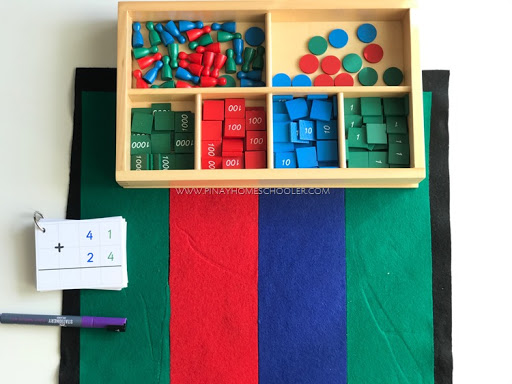What is Montessori Stamp Game?

In the album that I am using, this material is introduced after the child works on the Golden Beads Addition.  This is similar to Golden Beads but instead of beads, the numerical quantity is represented by stamps:  unit = unit stamps (green), ten beads = ten stamps (blue), hundred beads = hundred stamps (red) and thousand beads = thousand stamps (green).

Note:  before you use the Stamp Game for mathematical work, you should have a basic presentation of forming quantities with this material similar to the Golden Beads.  This basic presentation is not part of the post though, but you will see this in our previous work here, Mastering the Decimal System.

Purpose

Though this learning material is used to reinforce all arithmetic concepts, in this post, I will only share how it is used in addition (static and dynamic).

For what age?

4 years and older.

Materials needed for this activity:

Static addition is the first step in learning addition.  This form of addition means that there’s no regrouping or exchanging of two quantities.  Below is an example of how static addition is done using the Stamp Game.

Using your addition command cards or equation slip, form the quantities in the equation.  As you can see we have 23 + 31.  In the first numeral, we have 2 tens and 3 units, select the appropriate stamps that represent this numeral and place them in your stamp game mat.  Next is 31 which has 3 tens and 1 unit, select again the appropriate stamps representing this number and place them just below the first numeral's stamps. Be sure to leave a space between the two numerals. (see image below for the demonstration)

Before proceeding, read the numerals written in the paper slip and read the numeral presented by the stamps.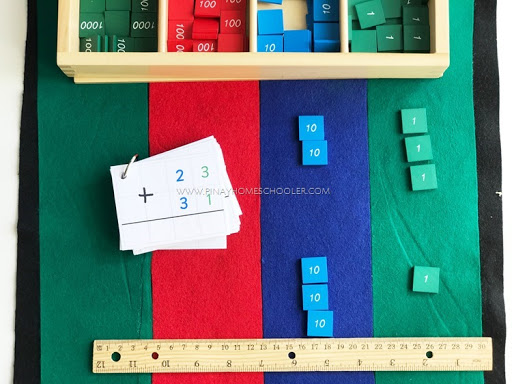To add the two numerals, push the rows of stamps together (combining the stamps) removing the space between the numerals.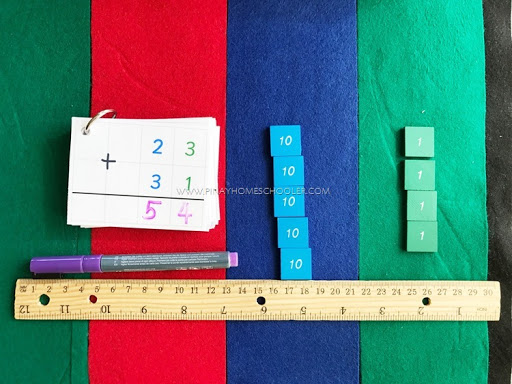Count the units first and record the number in your command card.  Then, count the tens and record the number in the card.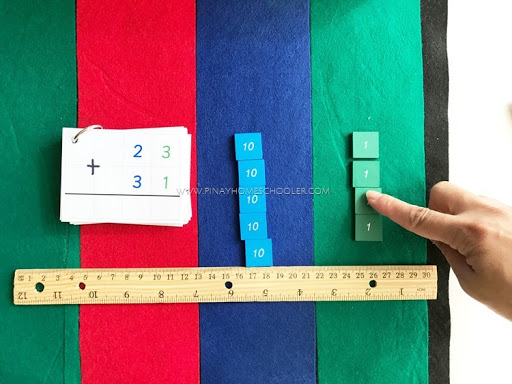Once the answer is written in the slip/equation card, read out loud the equation once again, together with its answer.Dynamic addition is used when the sum of two numerals is more than 10, thus regrouping is implemented.  This is the second lesson in addition once the child has a solid foundation in static addition.

The first step is to form the quantities in the addition command card.  Here you can see we have the numbers 35 (3 tens and 5 units) and 27 (2 tens and 7 units).  Again, make sure to add a space between the two numbers.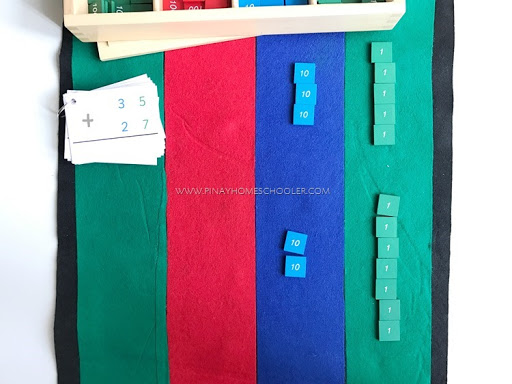Add the units place value first.  Here you will see that we have a total of 12 for the units place value, which is more than 10 thus a regrouping should be done.  Count the units and when you reach ten, ask the child “What does ten units make?”  The child should say “one ten”.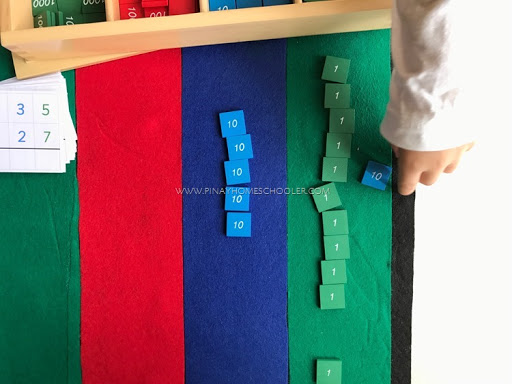Now regroup the ten units place value for one ten, placing the newly formed one ten to the tens column or place value, leaving 2 units in the units column.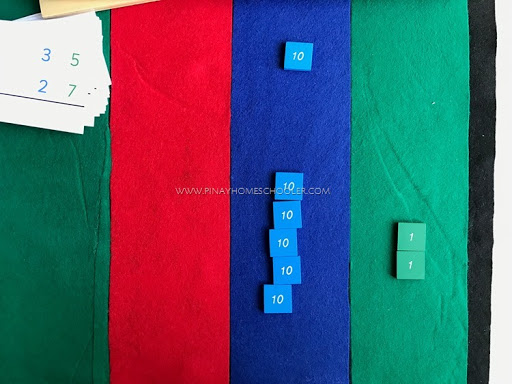From here, you add the tens.  If it is more than ten tiles, perform the same method of regrouping as explained previously (exchanging ten tiles of tens to one hundred tile).  But in our example, we only have 6 tens, thus no regrouping is needed.  Count the tens, and record the answer to your command slip.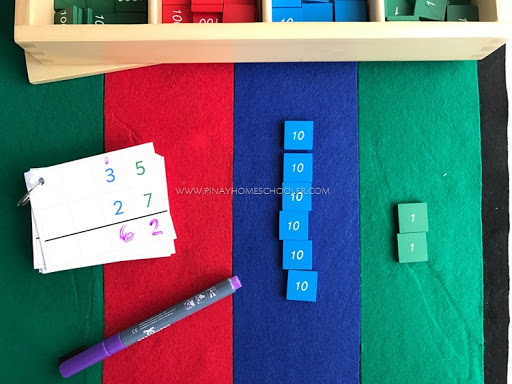For a VIDEO DEMO of the process you can watch our video here, Addition Using the Montessori Stamp Game.

You can find some old posts of Mavi working on the stamp game here.